Courses

# Test: Electrochemical Series

## 28 Questions MCQ Test Chemistry for JEE | Test: Electrochemical Series

Description
This mock test of Test: Electrochemical Series for JEE helps you for every JEE entrance exam. This contains 28 Multiple Choice Questions for JEE Test: Electrochemical Series (mcq) to study with solutions a complete question bank. The solved questions answers in this Test: Electrochemical Series quiz give you a good mix of easy questions and tough questions. JEE students definitely take this Test: Electrochemical Series exercise for a better result in the exam. You can find other Test: Electrochemical Series extra questions, long questions & short questions for JEE on EduRev as well by searching above.
QUESTION: 1

### Only One Option Correct Type This section contains multiple choice questions. Each question has four choices (a), (b), (c) and (d), out of which ONLY ONE is correct. Q. Cl2 gas is passed into a solution containing KF, Kl and KBr, and CHCI3 is added. There is a colour in CHCI3 (lower) layer. It is due to

Solution:

Based on electro chemical series, oxidising power of
F2 > Cl2 > Br2 > I2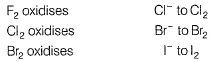On passing Cl2 in to a solution containing KF, Kl and KBr,
2KBr + Cl2 → 2KCI + Br2 (orange)
2KI + Cl→ 2KCI + l2 (violet)
2KI + Br2 → 2KBr + l2 (violet)
Br2 formed also oxidises Kl to l2 (violet)

QUESTION: 2

### Select the correct statement(s) about galvanic cell (Daniell cell) with E° cell​ = 1.10 V

Solution:

E° cell = 1.10 V
(a) Hence , when Eext < 1.10 V, reaction continues till the Eext reaches 1.10 V and
Zn(s) + Cu2+(aq) → Cu(s) + Zn2+(aq)
thus , zinc dissolves at anode and Cu deposits at cathode.
(b) When Eext = 1.10 V , no reaction.
(c) When Eext > 1.10V, reverse reaction takes place,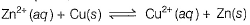It now acts as electrolytic cell.
Zinc deposits at zinc plate which is now the cathode (+ ve plate) and Cu dissolves as Cu2+ at copper plate which is now the anode (- ve plate)

QUESTION: 3

### Given,  E° Ag+/Ag = 0.80 V E° Mg2+/Mg = -2.37 V E° Cu2+/Cu = + 0.34V  E° Hg2+/Hg = 0.79V Q. Which of the following statement(s) is/are correct?

Solution:

Based on electro chemical series, reactivity series is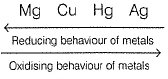(a) 2Ag+ + Cu → Cu2+ 2Ag
Cu is a better reducing agent than Ag hence Ag+ is reduced by Cu. Thus AgNO3 can not be stored in copper vessel.
(b) Cu2+ + Mg → Mg2+ + Cu
Mg will reduce Cu2+ to Cu thus can not be stored.
(c) Cu2+ + 2Ag → Cu + 2 Ag+
Cu is better reducing agent hence CuCI2 can be stored in silver vessel.
(d) Hg2+ + Cu → Cu2+ + Hg
Cu is better reducing agent. Hence, HgCI2 can not be stored in copper vessel.

QUESTION: 4

One mole of Ag2CO3 is strongly heated in an open vessel. Residue wll be

Solution: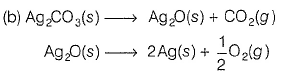Oxides of the metals are decomposed to metals if in electro chemical series , Eored > 0 .7 9 V

For A g, E°Ag+/Ag = 0.80 V hence Ag2O is decomposed to metals. Products are Ag , CO2 and O2. Since reaction takes place in an open vessel, CO2 and O2 escape from mixture, residue being 2 moles of silver.

QUESTION: 5

Zinc ,silver and iron plates are dipped in CuSO4 solutions placed in different vessels as shown: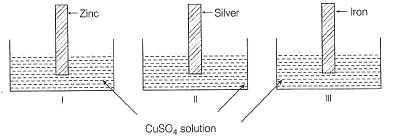Blue colour of CuSO4 fades in

Solution:

Blue colour fades or changes to colourless if Cu2+ is reduced to Cu.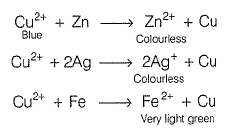In electrochemical series (ECS), reactivity order is Zn, Fe, Cu, Ag

Thus, Zn and Fe reduce Cu2+ to Cu but Ag does not reduce Cu2+. Thus, in I and III, CuSO4 changes to colourless or fades by zinc and iron plates.

QUESTION: 6

For the following half -cell reactions ,E° values are:

Mn2+(aq) + 2H2O(l)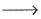MnO2(s) + 4H+(aq) + 2e-, E0 = -1.23V

MnO-4 (aq) + 4H+(aq) +3e-MnO2(s) + 2H2O(l), E0 = +1.70 V

Thus

Solution:

3Mn2+(aq) + 2MnO4-(aq)+ 2H2O (/) → 5MnO2(s) + 4H+  E°cell = 0.47 V
Since E°cell > 0, hence spontaneous.
Thus, Mn2+ is oxidised to MnO2 by MnO4- in acidic medium.

QUESTION: 7

Which of the following statement is correct?

Solution:

cell  remains unchanged on division , thus intensive property.

ΔrG is dependent on the division , thus extensive property.

QUESTION: 8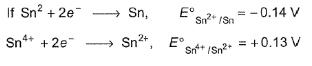Then

Solution: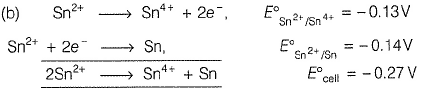Since E° cell < 0, disproportionation of Sn2+ to Sn4+ (by oxidation) and Sn (by reduction) is not spontaneous.

QUESTION: 9

Consider some facts about Standard Hydrogen Electrode(SHE).

I. It is assigned a zero potential at all temperature corresponding to the reaction.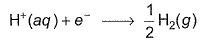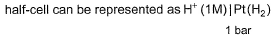II. Platinum electrode coated with platinum black is dipped in acidic solution and H2 gas is bubbled through it.
III. Concentration of both are oxidised and reduced forms of hydrogen is maintained at unity.

Select the correct facts

Solution: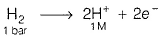It is oxidation half-cell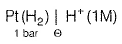H2 (reduced part) is at 1 bar. H+ (oxidised part) is at 1 M.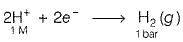It is reduction half-cell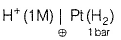In this case also, oxidised and reduced parts are at unity.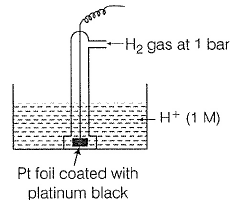Then (I), (II) and (III) are correct facts.

QUESTION: 10

The gas X at 1 atm is bubbled through a solution containing a mixture of 1 M Y- and 1 M Z- at 298 K.If the standard reduction potential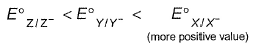then,

Solution: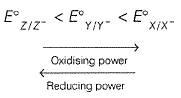In ECS, pair with more negative values of E°red reducing agent is above oxidising agent.
Thus, Z/Z- is the best reducing agent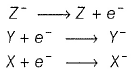Thus, Z- will reduce both X and Y and itself will be oxidised to Z .

QUESTION: 11

The standard reduction potential values of three metallic cations X, Y, Z are 0.52, -3.03 and -1.18 V respectively. The order of reducing power of the corresponding metals is

Solution:

x = - 0.52 V
y = -3.03 V
z = - 1.18 V

Their placements in ECS is in order Y > Z > X.
Thus, reducing nature is also in same order y > Z > X

QUESTION: 12

A solution containing one mole per litre each of Cu(NO3)2 ,AgNO3 ,Hg2(NO3)2 and Mg(NO3)is being electrolysed using inert electrodes. The value of standard redution potentials are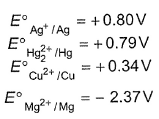with increasing voltage , the sequence of deposition of metals on the cathode will be

Solution:

These metals based on E°red values are placed in the following order: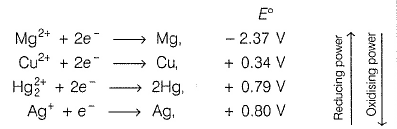Mg will reduce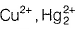and Ag+ to metals Cu, Hg, Ag.
Cu will reduce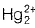and Ag+ to Hg, Ag, Hg will reduce Ag+ to Ag.
Ag+ is reduced by all metals thus Ag first. Hg2+ is reduced by Cu, Mg thus Hg. Cu2+ is reduced by Mg only, thus Cu.
Thus, deposition :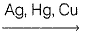QUESTION: 13

The standard reduction potentials at 298K for the following half-cell are given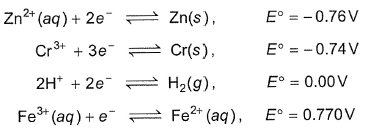Which is the strongest reducing agent?

Solution:

In electrochemical series (ECS), elements have been arranged in the increasing standard reduction potential starting from most negative to most positive value w.r.t. SHE (E°she= 0.00 V).

Most negative standard reduction potential means most easily oxidised and thus is the best reducing agent.

OR Reducing agent in (ECS) is always above oxidising agent. Thus, Zn(s) is the best reducing agent in given set.

QUESTION: 14

The Eo M3+/M2+ values for Cr, Mn, Fe and Co are - 0.41 V, +1,57 V, + 0.77 V and m /m 1.97 V respectively. For which one of these metals the change in oxidation state from +2 to +3 is easiest?

Solution: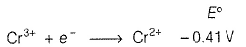Mn3+ + e- → Mn2+ + 1.57 V
Fe3+ + e- → Fe2+ + 0.77 V
Co3+ + e- → Co2+ + 1.97

Cr3+/Cr2+ with most negative E°red is the best reducing agent.
Cr2+→ Cr3+ + e- , E° = 0.41 V
Thus, is oxidised from Cr2+ to Cr3+ most easily.

QUESTION: 15

Given the standard electrode potentials

I. K+/K = -2.93V,
II. Ag+/Ag = 0.80V,
III. Hg2+/Hg = 0.79 V
IV. Mg2+/Mg = -2.37V,
V. Cr3+/Cr = - 0.74 V

These metals are arranged in increasing reducing power as

Solution:

Most negative E°red means the Mn+/M is at the top of ECS and is the best reducing agent.

QUESTION: 16

Statement Type

Direction : This section is based on Statement I and Statement II. Select the correct answer from the codes given below.

Statement I : CuSO4 can be stored in a vessel made of zinc.

Statement II :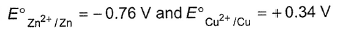w.r.t SHE

Solution:

Zn2+/Zn = -0.76 V
Cu2+/Cu = + 0.34 V

In electrochemical series, zinc is above copper and thus zinc is a better reducing agent than copper. When CuS04 is placed in zinc vessel, copper is displaced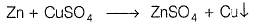Thus, CuSO4 cannot be stored in a vessel made of zinc.
Thus, statement I is incorrect and statement II is correct.

QUESTION: 17

Statement I​ : When AgNO3 solution is stirred with a spoon made of copper,solution turns blue.

Statement II : In electrochemical series ,copper is above silver

Solution: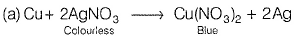In electrochemical series, copper is above silver thus is a better reducing agent. When AgNO3 solution is stirred with copper spoon, Ag is displaced and copper is oxidised to Cu2+ (blue).

Thus, statement I and II are correct and statement II is the correct explanation of statement I.

*Multiple options can be correct
QUESTION: 18

The positive value of the standard electrode potential of Cu2+/Cu,

(E°Cu2+/Cu = 0.34 V) indicates that

Solution: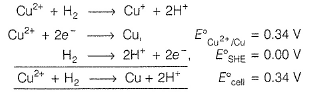cell > 0 thus spontaneous
Cu2+/Cu couple is thus a stronger oxidising agent. Reverse reaction is non-spontaneous.
Cu + 2H+ → Cu2+ + H2, E°cell = - 0.34 V
Thus, copper cannot displace H2 from acid.

*Multiple options can be correct
QUESTION: 19

Consider the following half-reactions: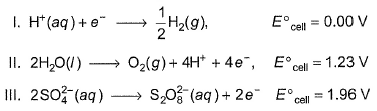Select the correct statements on the basis of the above data

Solution: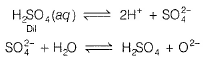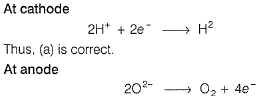Thus, (c) is correct.
Concentrated H2SO4 ionises as H+ and HSO4- and then water is not oxidised in concentrated H2SO4 Thus, (b) is incorrect.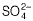is not oxidised to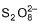in dilute H2SO4. Thus, (d) is incorrect.
(d) In dilute sulphuric acid solution,ions will be oxidised to tetrathionate ion () at anode.

*Multiple options can be correct
QUESTION: 20

Select the correct statement(s) based on the following half-reaction: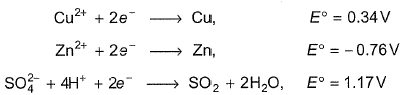Solution:

If dil. H2SO4 is used, then H+ is the reacting species.
If cone. H2SO4 is used, then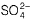is the reacting species.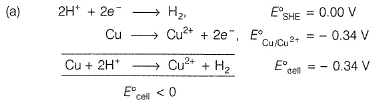Thus, copper does not react with H+ (dil. H2SO4) forming H2.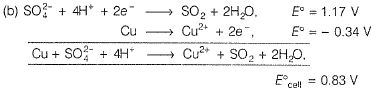Since E°cell > 0, Thus copper reacts with cone. H2SO4 forming SO2.
(c) Zn ++4H+ → Zn2+ + SO2 + 2H2O
cell = 1.93 V, E°cell > 0, thus zinc also reacts with conc.
H2SO4 forming SO2.
(d) Zn + 2H+ → Zn2+ + H2, E°cell = 0.76 V
cell > 0, thus zinc reacts with dil. H2SO4 forming H2.

QUESTION: 21

Matching List Type

Choices for the correct combination of elements from Column I and Column II are given as options (a), (b), (c) and (d), out of which one is correct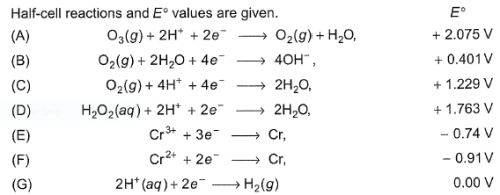Match the half-cell reaction in Column I with their values in Column II and select the answer from the answer codes at the end.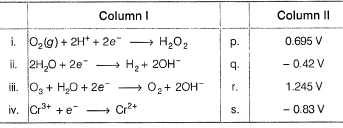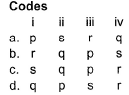Solution: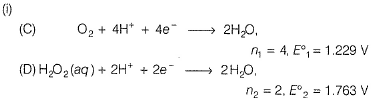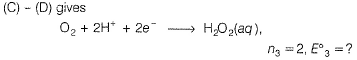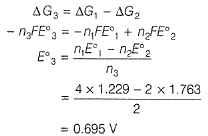Thus, (i) → (p)
(ii) (B) O2(g) + 2H2O + 4e- → 40H- n1 = 4, E1 = 0.401 V
(C) O2(g)+4H+ 4e- → 2H2O , n2 = 4, E2 = 1.229 V
(G) 4H+ + 4e- → 2H2 , n3 = 4, E3 = 0.00 V
(B) + (G) - (C) gives
4 H2O + 4e- → 2H2 + 40H-
2 H2O + 2e- → H2 + 2OH-
∴ E4 = 0.401 + 0.00- 1.229
= -0.83 V
Thus, (ii) → (s)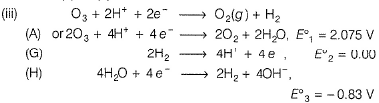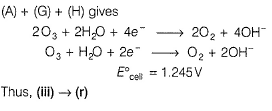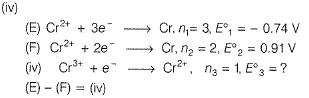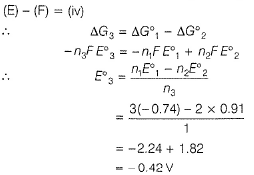Thus, (iv) → (s)

QUESTION: 22

Passage I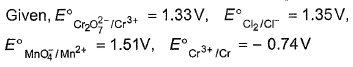The most stable oxidised species is

Solution: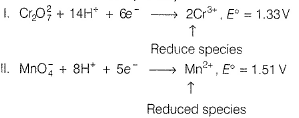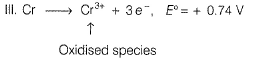For (I) and (II), E° > 0, but for reduced species.
For (III), E° > 0, thus Cr3+ is the most stable.
More negative value of standard electrode reduction potential, better the reducing agent. Thus,
Mn2+ < CI- < Cr3+ < Cr

QUESTION: 23

Passage IReducing power of different species is in the order

Solution:For (I) and (II), E° > 0, but for reduced species.
For (III), E° > 0, thus Cr3+ is the most stable.
More negative value of standard electrode reduction potential, better the reducing agent. Thus,
Mn2+ < CI- < Cr3+ < Cr

QUESTION: 24

Passage II

Accidently chewing of a stray fragment of aluminium foil can cause a sharp tooth pain if the aluminium comes in contact with an amalgam filling. This filling, an alloy of silver, tin and mercury acts as the cathode (of a tiny galvanic cell), the aluminium as anode and saliva (of mouth) serves as electrolyte. When the aluminium and the filling come in contact, an electric current passes from aluminium to the filling, which is sensed by a nerve in the teeth.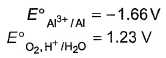Q.

Net reaction taking place when amalgam is in contact with aluminium foil is

Solution: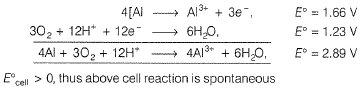QUESTION: 25

Passage II

Accidently chewing of a stray fragment of aluminium foil can cause a sharp tooth pain if the aluminium comes in contact with an amalgam filling. This filling, an alloy of silver, tin and mercury acts as the cathode (of a tiny galvanic cell), the aluminium as anode and saliva (of mouth) serves as electrolyte. When the aluminium and the filling come in contact, an electric current passes from aluminium to the filling, which is sensed by a nerve in the teeth.Q.

Standard emf experienced by the person with dental filling is

Solution: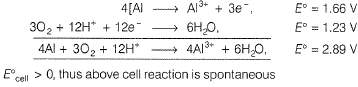QUESTION: 26

Passage III

When suspected drunk drivers are tested with a breathalyser, the alcohol (ethanol) in the exhaled breath is oxidised to ethanoic acid with an acidic solution of potassium dichromate.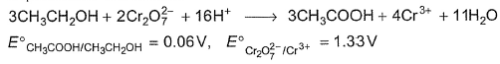Q.

Under standard state, E°cell of the breathalyser would be

Solution:

CH3CH2OH is oxidised to CH3COOH and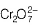is reduced to Cr3+.
cell - E°CH3CH2OH/CH3COOH + E°/Cr3+
= -0.06+1.33
= 1.27 V
Reaction quotient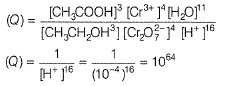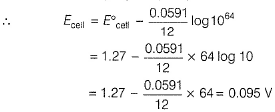QUESTION: 27

Passage III

When suspected drunk drivers are tested with a breathalyser, the alcohol (ethanol) in the exhaled breath is oxidised to ethanoic acid with an acidic solution of potassium dichromate.Q.

Under the condition of unit activity of each species,emf recorded in the breathalyser at pH 4 would be

Solution:

CH3CH2OH is oxidised to CH3COOH andis reduced to Cr3+.
cell - E°CH3CH2OH/CH3COOH + E°/Cr3+
= -0.06+1.33
= 1.27 V
Reaction quotient*Answer can only contain numeric values
QUESTION: 28

E° values of Mn+ + ne- → M are given below
Fe2+/Fe -0.44 V, Mg2+/Mg -2.37 V, Pt(H2)/H+ 0.00 V,
AI3+/AI -1.66 V , Zn2+/Zn - 0.76 V, Cu2+/Cu +0.34 V,
Ag+/Ag + 0.80 V, Ni2+/Ni -0.26 V

How many of the metals can be used to prevent corrosion of Ni to Ni2+?

Solution:

Fe, Al, Zn and Mg can be used to protect Ni from being oxidised to Ni2+ since, E°cell > 0 and ΔG° < 0.